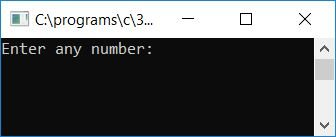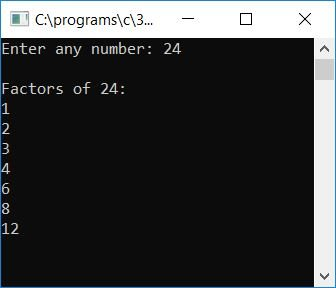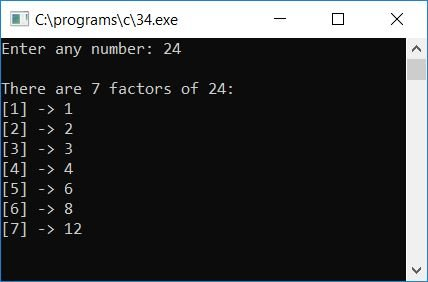# C Program to Find and Print All Factors of a Given Number

In this article, we will learn how to create a program in C that will ask the user to enter any number (at run-time) as input and find and print all the factors of that given number as output. Here is the program:

```#include<stdio.h>
#include<conio.h>
int main()
{
int num, rem, i;
printf("Enter any number: ");
scanf("%d", &num);
printf("\nFactors of %d:\n", num);
for(i=1; i<num; i++)
{
if(num%i==0)
printf("%d\n", i);
}
getch();
return 0;
}```

The program was written in the Code::Blocks IDE, and here is the sample run after a successful build and run. This is the first snapshot of the sample run:Supply any number, say 24, to see all the factors of 24 after pressing the ENTER key:Here are some of the main steps used in the above program:

• Receive any number as input, say 24.
• Create a for loop that runs from 1 to one less than the number, say 23 here (if input is 24).
• Inside the for loop, use the if block to apply the divisibility test of 24 to each and every number from 1 to 23.
• That is, if any of the numbers totally divide the given number, say 24, then print it out as a factor of the given number and continue to check for the next number.
• i is set to 1 during the first run of the for loop. 1 totally divides 24 without leaving any remainder; therefore, 1 will be one of the factors of 24.
• At the second run of the for loop, i holds 2. 2 totally divides 24 without leaving any remainder; therefore, 2 will also be one of the factors of 24.
• i hold 3 again on the third run. It also divides 24 without leaving any remainder, so 3 will also be one of the factors of 24.
• In the same way, check for all the numbers up to 23 and print them out if the number totally divides the given number, say 24, without leaving any remainder.

Now let's modify the above program in such a way that the program will print the total number of factors in a number-wise list. Here is the program. The question is: write a program in C to print all the factors of any given number given by the user at run-time. For example, if the user has provided 24 as input, The output must then look like this:

```There are 7 factors of 24:
 -> 1
 -> 2
 -> 3
 -> 4
 -> 6
 -> 8
 -> 12```

The program given below is the answer to the above question:

```#include<stdio.h>
#include<conio.h>
int main()
{
int num, rem, i, count=0;
printf("Enter any number: ");
scanf("%d", &num);
for(i=1; i<num; i++)
{
if(num%i==0)
count++;
}
printf("\nThere are %d factors of %d:\n", count, num);
count=1;
for(i=1; i<num; i++)
{
if(num%i==0)
{
printf("[%d] -> %d\n", count, i);
count++;
}
}
getch();
return 0;
}```

The following is the final snapshot of the above program's sample run:C Quiz

« Previous Program Next Program »